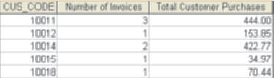# Write a query to produce the number of invoices and the total purchase amounts by customer, using the output shown in Figure P7.18 as your guide. Note the results are sorted by customer code. (Compare this summary to the results shown in Problem 17.) FIGURE P7.18 NUMBER OF INVOICES AND TOTAL PURCHASE AMOUNTS BY CUSTOMER### Database Systems: Design, Implemen...

13th Edition
Carlos Coronel + 1 other
Publisher: Cengage Learning
ISBN: 9781337627900

#### Solutions

Chapter
Section### Database Systems: Design, Implemen...

13th Edition
Carlos Coronel + 1 other
Publisher: Cengage Learning
ISBN: 9781337627900
Chapter 7, Problem 18P
Textbook Problem
118 views

## Write a query to produce the number of invoices and the total purchase amounts by customer, using the output shown in Figure P7.18 as your guide. Note the results are sorted by customer code. (Compare this summary to the results shown in Problem 17.)FIGURE P7.18 NUMBER OF INVOICES AND TOTAL PURCHASE AMOUNTS BY CUSTOMERProgram Plan Intro

“GROUP BY” Clause:

The GROUP BY clause is used to group the result of a SELECT statement done on a table where the tuple values are similar for more than one column.

Syntax:

SELECT expression1, expression2, expression_n, aggregate_function (expression)FROM table_name WHERE conditions GROUP BY expression1, expression2, expression_n;

Aggregate Functions:

SQL(Structured Query Language) has some built-in functions and they are called as aggregate functions. SQL contains five built-in functions. They are:

• SUM – This function is used to add values from the particular column.
• Syntax: SELECT SUM(column_Name) FROM table_Name;
• COUNT – This is used to count the number of rows for the particular column.
• Syntax: SELECT COUNT(column_Name) FROM table_Name;
• MAX – This function is used to get the maximum value from the column.
• Syntax: SELECT MAX(column_Name) FROM table_Name;
• MIN – This function is used to get the minimum value from the column.
• Syntax: SELECT MIN(column_Name) FROM table_Name;
• AVG – This function is used to get the average of all the values from the column.
• Syntax: SELECT AVG(column_Name) FROM table_Name;

### Explanation of Solution

SQL code:

The SQL code is to produce the number of invoices, total purchase amounts that sorted by customer code is given below:

SELECT DISTINCT ROW [Chapter 7 Problem 17].CUS_CODE AS CUS_CODE, Count([Chapter 7 Problem 17].INV_NUMBER) AS CountOfINV_NUMBER, Sum([Chapter 7 Problem 17].Expr1) AS SumOfExpr1

FROM [Chapter 7 Problem 17]

GROUP BY [Chapter 7 Problem 17].CUS_CODE;

Here, the “[Chapter 7 Problem 17]” refer the following code and the code is saved as “[Chapter 7 Problem 17]”.

SELECT DISTINCTROW INVOICE.CUS_CODE, LINE.INV_NUMBER, Sum([LINE]![LINE_UNITS]*[LINE]![LINE_PRICE]) AS Expr1

FROM INVOICE INNER JOIN LINE ON INVOICE.INV_NUMBER = LINE.INV_NUMBER

GROUP BY INVOICE.CUS_CODE, LINE.INV_NUMBER;

The above code displays the following result:

Screenshot of the table

### Still sussing out bartleby?

Check out a sample textbook solution.

See a sample solution

#### The Solution to Your Study Problems

Bartleby provides explanations to thousands of textbook problems written by our experts, many with advanced degrees!

Get Started

Find more solutions based on key concepts
Name at least four fundamental dimensions.

Engineering Fundamentals: An Introduction to Engineering (MindTap Course List)

What are control relays?

Electric Motor Control

Suspicious File Attachment You receive an email message that appears to be from someone you know. When you try ...

Enhanced Discovering Computers 2017 (Shelly Cashman Series) (MindTap Course List)

If your motherboard supports ECC DDR3 memory, can you substitute non-ECC DDR3 memory?

A+ Guide to Hardware (Standalone Book) (MindTap Course List)

What are the four phases in the social engineering attack cycle?

Network+ Guide to Networks (MindTap Course List)

What types of tip seals are used with cutting torch tips?

Welding: Principles and Applications (MindTap Course List)